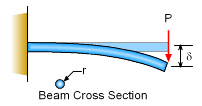Ch 6. Modeling and Similitude Multimedia Engineering Fluids DimensionalAnalysis Similitude DimensionlessGroups
 Chapter 1. Basics 2. Fluid Statics 3. Kinematics 4. Laws (Integral) 5. Laws (Diff.) 6. Modeling/Similitude 7. Inviscid 8. Viscous 9. External Flow 10. Open-Channel Appendix Basic Math Units Basic Equations Water/Air Tables Sections Search eBooks Dynamics Fluids Math Mechanics Statics Thermodynamics Author(s): Chean Chin Ngo Kurt Gramoll ©Kurt GramollFLUID MECHANICS - CASE STUDY IntroductionDeflection of a Cantilever Beam To illustrate that dimensional analysis introduced in fluid mechanics can also be used in other engineering problems (e.g., statics, dynamics and solid mechanics), students are challenged to find the important dimensionless parameters governing the deflection of a circular cantilever beam due to an applied force at its tip, as shown in the figure. Questions Determine the dimensionless parameters involved using dimensional analysis. Approach First, list all the parameters of the problem. Hint: One of the parameters is the modulus of elasticity (E) , which is a material property of the beam. Determine the number of basic dimensions involved. Find the number of pi terms using Buckingham Pi theorem. Determine the pi terms.

Practice Homework and Test problems now available in the 'Eng Fluids' mobile app
Includes over 250 problems with complete detailed solutions.
Available now at the Google Play Store and Apple App Store.